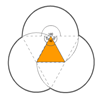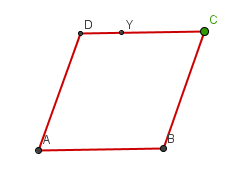#### You may also like### Just Rolling Round

P is a point on the circumference of a circle radius r which rolls, without slipping, inside a circle of radius 2r. What is the locus of P?### Rolling Coins

A blue coin rolls round two yellow coins which touch. The coins are the same size. How many revolutions does the blue coin make when it rolls all the way round the yellow coins? Investigate for a line of 'n' coins### Triangles and Petals

An equilateral triangle rotates around regular polygons and produces an outline like a flower. What are the perimeters of the different flowers?

# Roaming Rhombus

##### Age 14 to 16 Challenge Level:

Four rods of equal lengths are hinged at their endpoints to form a rhombus ABCD.Keeping AB fixed, CD is free to move in the plane with the angle BAD between 0 and 180 degrees.

What is the locus (or path) of the point D?

What is the locus of Y, the point one third of the way along DC? (DY:YC = 1:2)